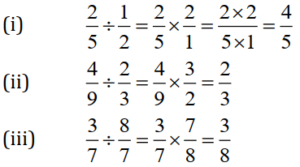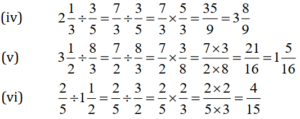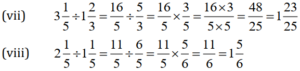# Free Ncert Solution for Class 7 Maths Chapter 2 Fractions and Decimals Exercise 2.3

Fractions and Decimals Class 7 Ex. 2.3;

New Ncert Solution for Class 7 Maths Chapter 2 Fractions and Decimals Free Solution;

Exercise 2.3

Question 1 :- Find: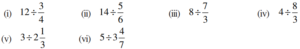Solution 1:-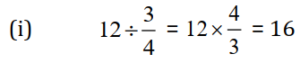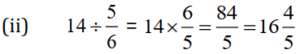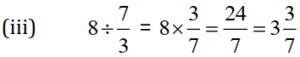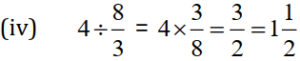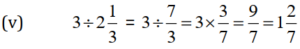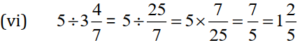Question 2 :- Find the reciprocal of each of the following fractions. Classify the reciprocals as proper fractions, improper fractions and whole numbers.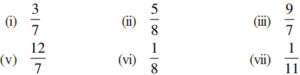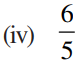Solutions 2:-

(i) Reciprocal of 3/7=7/3, which is improper fraction.
(ii) Reciprocal of 5/8=8/5, which is improper fraction.
(iii) Reciprocal of 9/7=7/9, which is proper fraction.
(iv) Reciprocal of 6/5=5/6, which is proper fraction.
(vi) Reciprocal of 12/7=7/12, which is proper fraction.
(vi) Reciprocal of 1/8=8/1, which is whole number.
(vii) Reciprocal of 1/11=11/1, which is whole number.

Question 3 :- Find: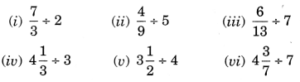Solution 3:-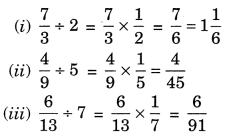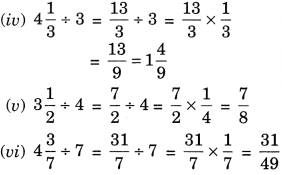Question 4 :- Find: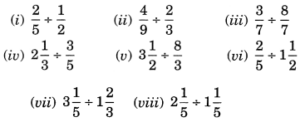Solution 4:-